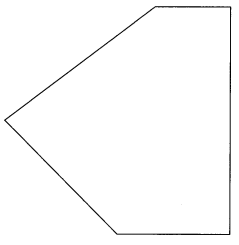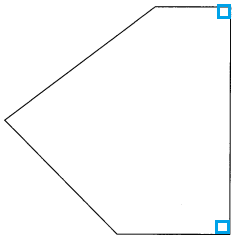# Math in Focus Grade 3 Chapter 17 Practice 2 Answer Key Right Angles

This handy Math in Focus Grade 3 Workbook Answer Key Chapter 17 Practice 2 Right Angles provides detailed solutions for the textbook questions.

## Math in Focus Grade 3 Chapter 17 Practice 2 Answer Key Right Angles

Look at these angles. Use a piece of folded paper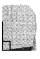to help you answer the questions.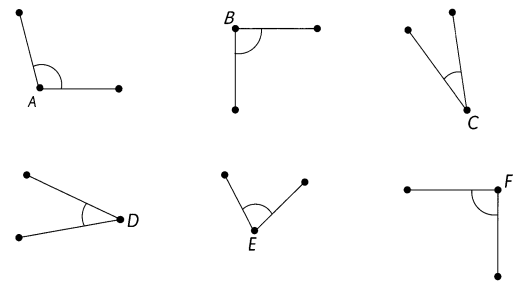Question 1.
Which angle is less than a right angle?
Angle ________________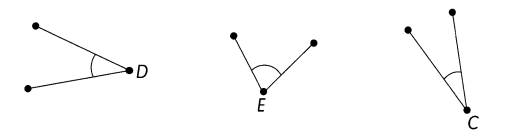Angle  C, D and E are angles less then a right angle.
Explanation:
Angle less than a right angles are Acute angles.

Question 2.
Which angle is greater than a right angle?
Angle ________________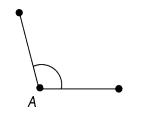Angle A is greater then a right angle.
Explanation:
Angle greater than a right angles are Obtuse angles.

Question 3.
Which angles are the same size as right angles?
Angles _________________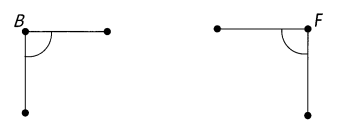Angle A and F are the same as right angles.
Explanation:
The angle bounded by two lines perpendicular to each other.
A right triangle has one angle exactly equal to 90 degrees.

Mark all the right angles in each figure.

Question 4.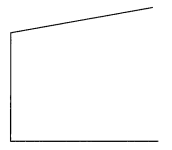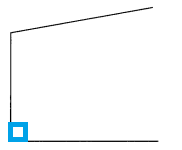Explanation:
The above figure has,
One right angle is there, which is marked blue color.
The angle bounded by two lines perpendicular to each other.
A right triangle has one angle exactly equal to 90 degrees.

Question 5.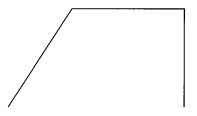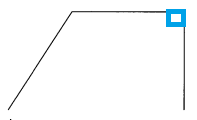Explanation:
The above figure has,
One right angle is there, which is marked blue color.
The angle bounded by two lines perpendicular to each other.
A right triangle has one angle exactly equal to 90 degrees.

Question 6.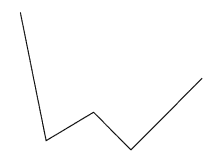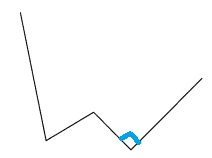Explanation:
The above figure has,
One right angle is there, which is marked blue color.
The angle bounded by two lines perpendicular to each other.
A right triangle has one angle exactly equal to 90 degrees.

Question 7.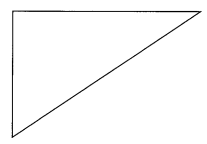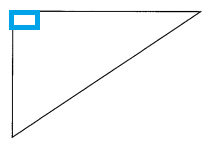Explanation:
The above figure has,
One right angle triangles , the angle is marked blue color.
The angle bounded by two lines perpendicular to each other.
A right triangle has one angle exactly equal to 90 degrees.

Question 8.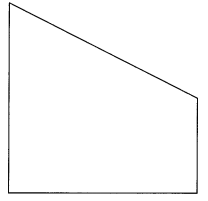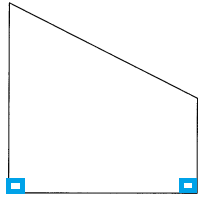Explanation:
The above figure has,
Two right angle are there, which are marked blue color.
The angle bounded by two lines perpendicular to each other.
A right triangle has one angle exactly equal to 90 degrees.

Question 9.